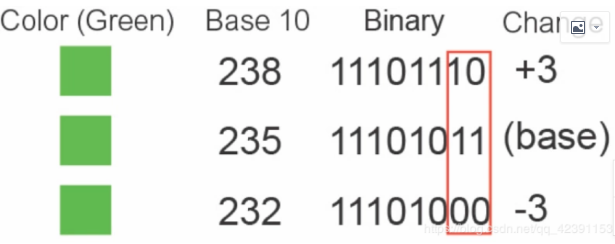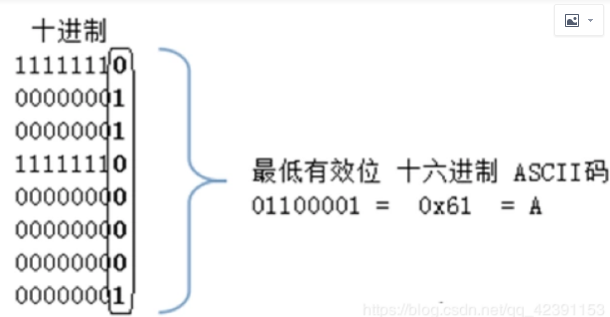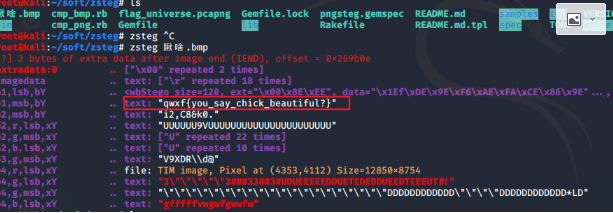关联规则 关联知识 关联工具 关联文档 关联抓包
 参考1(官网) 参考2 参考3

[SAFE-ID: JIWO-2020-2592]   作者: future 发表于: [2020-02-28]

LSB图片隐写

LSB全称为 least significant bit，是最低有效位的意思。Lsb图片隐写是基于lsb算法的一种图片隐写术。LSB图片隐写的检测工具zsteg安装说明连接如下

binwalk无果winhex无果# -*- coding: UTF-8 -*-
from PIL import Image
def plus(str):
#Python zfill() 方法返回指定长度的字符串，原字符串右对齐，前面填充0。
return str.zfill(8)
def get_key(strr):
#获取要隐藏的文件内容
tmp = strr
f = file(tmp,"rb")
str = ""
for i in range(len(s)):
#逐个字节将要隐藏的文件内容转换为二进制，并拼接起来
#1.先用ord()函数将s的内容逐个转换为ascii码
#2.使用bin()函数将十进制的ascii码转换为二进制
#3.由于bin()函数转换二进制后，二进制字符串的前面会有"0b"来表示这个字符串是二进制形式，所以用replace()替换为空
#4.又由于ascii码转换二进制后是七位，而正常情况下每个字符由8位二进制组成，所以使用自定义函数plus将其填充为8位
str = str+plus(bin(ord(s[i])).replace('0b',''))
#print str
f.closed
return str
def mod(x,y):
return x%y;
#str1为载体图片路径，str2为隐写文件，str3为加密图片保存的路径
def func(str1,str2,str3):
im = Image.open(str1)
#获取图片的宽和高
width = im.size
print "width:"+str(width)+"\n"
height = im.size
print "height:"+str(height)+"\n"
count = 0
#获取需要隐藏的信息
key = get_key(str2)
keylen = len(key)
for h in range(0,height):
for w in range(0,width):
pixel = im.getpixel((w,h))
a=pixel
b=pixel
c=pixel
if count == keylen:
break
#下面的操作是将信息隐藏进去
#分别将每个像素点的RGB值余2，这样可以去掉最低位的值
#再从需要隐藏的信息中取出一位，转换为整型
#两值相加，就把信息隐藏起来了
a= a-mod(a,2)+int(key[count])
count+=1
if count == keylen:
im.putpixel((w,h),(a,b,c))
break
b =b-mod(b,2)+int(key[count])
count+=1
if count == keylen:
im.putpixel((w,h),(a,b,c))
break
c= c-mod(c,2)+int(key[count])
count+=1
if count == keylen:
im.putpixel((w,h),(a,b,c))
break
if count % 3 == 0:
im.putpixel((w,h),(a,b,c))
im.save(str3)
#原图
old = "E:\Users\\test.png"
#处理后输出的图片路径
new = "E:\Users\\LSBencode.png"
#需要隐藏的信息
enc = "E:\Users\\flag.txt"
func(old,enc,new)

### 评论

##### 发表评论顶 踩